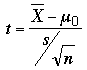SEARCH HOMEMath Central Quandaries & QueriesQuestion from Rachel, a student: Hi, I am wanting to use the t-test to find if temperature over a period of 5 days was significantly different to the mean temperature for that time of year. So i want to compare expected temps to actual temps. The problem is that because the expected temperature is the same for the 5 days it gives me a standard deviation of zero. Does this mean the t-test cannot be used because the f-test can't be calculated. If so is there an alternative test? Thanks very much!! RachelHi Rachel,

It looks like you are attempting to apply a two sample t-test where you only have one sample. You know the expected temperature μ0 and you want to test to see if the mean temperature for n = 5 days is significantly different than μ0. You can test this hypothesis using the test statisticThis statistic has the t-distribution with n - 1 = 4 degrees of freedom.

HarleyMath Central is supported by the University of Regina and The Pacific Institute for the Mathematical Sciences.